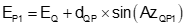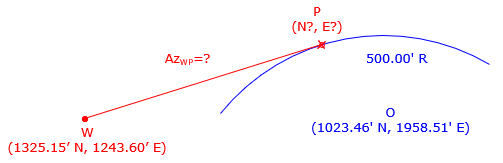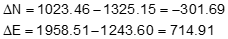This chapter explains several curve and tangent line-curve fitting situations.  They can be used with other COGO tools to construct complex curvilinear traverses having to fit specific mathematical conditions.

### A. Fitting arc through three points

Three non-linear points define a circular arc, Figure I-1Figure I-1 Three point arc

If the coordinates of the three points (1, 2, and 3) are known, the arc radius (R) and radius point (O) coordinates can be determined.

Equations I-1 and I-2 are used to compute the radius point coordinates.Equation I-1Equation I-2

The coefficients for Equation I-1 are:Equation I-3Equation I-4Equation I-5Equation I-6

Once the radius point coordinates are determined, the arc radius can be computed from Equation I-7 .Equation I-7

Point i is any of the three points used to define the arc.

### B. Tangent line-arc intersection

The next two solutions deal with tangent a tangent line and arc.

Known information

• Coordinates at point Q
• Direction from point Q
• Coordinates of radius point, point O

Determine

• Coordinates of tangent point PFigure I-2 Radius determination

Solution process

Inverse along the line from point Q to the radius point, O, to determine the direction and length of the line.

Compute angle Q as the difference between the directions AzQO and AzQP, subtracting the smaller from the larger, depending on which side of line QO line QP is.

Solve the following equationsEquation I-8Equation I-9Equation I-10Equation I-11

Known information

• Coordinates of radius point, point O
• Coordinates of point Q

Determine

• Direction of a line from point Q tangent to the arc; there are two possible directions, compute both
• Coordinates of the two tangent points P1 and P2

Determine the direction of a line tangent to an arc.Figure I-3 Tangent direction determination

Solution process

Inverse along the line from point point Q to the radius point O to determine its direction and length.

Compute angle g using Equation I-12.Equation I-12

Angle g is used with the direction of line point Q to radius point O to determine the direction from point Q to each intersection point.

The distance from point Q to each intersection point is:Equation I-13

To compute the coordinates of P1, perform a Forward Computation from point Q:Equation I-13Equation I-14Equation I-15

To compute the coordinates of point P2, perform a Forward Computation from  point Q:Equation I-16Equation I-17Equation I-18

### C. Three-tangent arc

Three non-colinear intersecting lines can serve as tangent lines to define a circle, Figure I-5.Figure I-5 Tangent to three lines

Figure I-6 shows the primary geometry of the three-tangent arc.Figure I-6 Geometry - curve to left

Figure I-7 shows geometry for a curver to the right, as defined by the tangent azimuths.Figure I-7 Geometry - curve to the right

Points I, J, and K are the tangent points. These divide the curve into two tangent arcs having the same radius with points G and H as their Points of Intersection (PI).

Solution process

Determine PIs, G and H, by direction-direction intersection. Inverse between them to obtain the distance, dGH.

Using the azimuths, compute deflection angles ΔG and ΔH at the PIs.

Compute curve radius using Equation I-19Equation I-19

R is positive for a curve to the right; negative for a curve to the left.

Distances dGJ and dGI are the tangent distances for the first curve; distances dHK and dHJ are the tangent distances for the second curve. To compute an arc's tangent distance use:Equation I-20

To compute the coordinates of the radius point

• Forward Computation from point G to point J using AzB and tangent distance dGJ
• Compute azimuth to the radius point,  AzJO, by adding (curve to right) or subtracting (curve to left) 90° to/from AzB.
• Forward Computation from point J to point O using AzJO and R.

The coordinates of the radius point can also be computed by Forward Computation sequences:

• Point G to point I to point O
• Point H to point J to point O
• Point H to point K to point O

### D. Examples

#### 1. Three point curve

Three known coordinate points are

 Point North (ft) East (ft) A 902.72 1751.91 B 870.76 1237.76 C 538.57 1293.16

Determine the radius of the circle passing through the points and coordinates of the radius point.

SketchCompute the coefficients using Equations I-3 through I-6Use the coefficients in Equations I-1 and I-2 to get radius point coordinatesUsing point ACheck using point BChecks within rounding.

#### 2. Tangent line

What is the direction of the line line tangent to the arc shown below? What are the coordinates of the intersection point?Inverse from point W to the radius point OUsing Equation I-12 compute the angle g between the line to the radius point and tangent line.Determine the azimuth and length of the tangent line

.Since the tangent line is left of the line to the radius point, to get its azimuth subtract g from AzWO' Equation, I-16.Its length comes from Equation I-13Forward Computation, Equations I-17 and I-18, to compute tangent point coordinatesTangent azimuth 72°45'42" Tangent pt coords 1501.00' N 1810.34' E

#### 3. Three-tangent arc

What is the radius of the arc that is tangent to the three line shown?Label the geometryEstablsh points G and H using direction-direction intersections (results shown, comps left to user)

 Point North East G 1849.672 1305.139 H 1735.850 1670.923

ΔG and ΔH from the azimuths (comps left to user)

 ΔG 63°05'03" ΔH 49°03'40"

Inverse Computation to obtain distance from point G to point H  (comps left to user): 383.084

Apply Equation I-19 to get the arc radiusTangent distance for first arc section (comps left to user): 219.721

Radius point coordinates (comps left to user): 1,442.592' N and 1,408.580' E